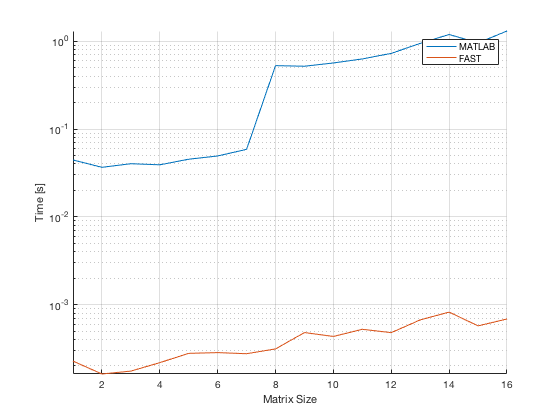## La Budde's Method For Computing Characteristic Polynomials

by Rizwana Rehman and Ilse C.F. Ipsen in arXiv, April 2011

Reproduce in Code (c) Sebastian Jiro Schlecht: Monday, 10. December 2018

```clear; clc; close all;
```

## Introduction

In this example, we reproduce a fast algorithm (Algorithm 2) to computer the characteristic polynomial of a square matrix and compare the output to the MATLAB build-in function charPoly.

```N = 12;
A = randn(N) + 1i*randn(N);
A = A / N;
disp('Comparison of Matlab charpoly and LaBudde charpoly')

%%-----------------
disp('Matlab')
tic
pMatlab = charpoly(A);
toc
disp(pMatlab)

%%-----------------
disp('LaBudde')
tic
pFast = fastCharPoly( A );
toc
disp(pFast)

%%compare
disp('Compare error')
disp(pMatlab - pFast)
```
```Comparison of Matlab charpoly and LaBudde charpoly
Matlab
Elapsed time is 0.729862 seconds.
Columns 1 through 4

1.0000 + 0.0000i  -0.3884 - 0.2123i   0.1163 + 0.0161i  -0.0139 + 0.0002i

Columns 5 through 8

0.0009 - 0.0046i   0.0027 + 0.0009i  -0.0004 - 0.0003i   0.0001 + 0.0003i

Columns 9 through 12

-0.0000 + 0.0001i  -0.0000 + 0.0000i  -0.0000 + 0.0000i  -0.0000 - 0.0000i

Column 13

-0.0000 + 0.0000i

LaBudde
Elapsed time is 0.000753 seconds.
Columns 1 through 4

1.0000 + 0.0000i  -0.3884 - 0.2123i   0.1163 + 0.0161i  -0.0139 + 0.0002i

Columns 5 through 8

0.0009 - 0.0046i   0.0027 + 0.0009i  -0.0004 - 0.0003i   0.0001 + 0.0003i

Columns 9 through 12

-0.0000 + 0.0001i  -0.0000 + 0.0000i  -0.0000 + 0.0000i  -0.0000 - 0.0000i

Column 13

-0.0000 + 0.0000i

Compare error
1.0e-15 *

Columns 1 through 4

0.0000 + 0.0000i   0.0555 - 0.1110i   0.0278 + 0.1249i  -0.0069 - 0.0304i

Columns 5 through 8

0.0159 - 0.0052i   0.0009 + 0.0011i   0.0005 - 0.0001i   0.0002 + 0.0001i

Columns 9 through 12

0.0000 + 0.0000i   0.0000 + 0.0000i  -0.0000 + 0.0000i  -0.0000 - 0.0000i

Column 13

-0.0000 + 0.0000i

```

## Speed comparison

We compare the performance of fastCharPoly to the MATLAB build-in function charPoly. It can be seen that fastCharPoly is more than 100 times faster.

```matrixSizes = 1:16;
numberOfRepetitions = 5;
performanceTime_MATLAB = zeros(length(matrixSizes),numberOfRepetitions);
performanceTime_FAST = zeros(length(matrixSizes),numberOfRepetitions);
for N = matrixSizes
% disp(matrixSize)
for repetition = 1:numberOfRepetitions
A = randn(N) + 1i*randn(N);
A = A / N;
tic
pMatlab = charpoly( A );
performanceTime_MATLAB(N,repetition) = toc;
tic
pFast = fastCharPoly( A );
performanceTime_FAST(N,repetition) = toc;

if max(abs(pMatlab - pFast)./pMatlab) < 1e-7
% disp('OK')
else
warning(['Results deviate by ' num2str(max(abs(pMatlab - pFast)))])
end
end
end
```

## Plot performance results

```averagePerformanceTime_MATLAB = mean(performanceTime_MATLAB,2);
averagePerformanceTime_FAST = mean(performanceTime_FAST,2);

figure(1); hold on; grid on;
plot(matrixSizes,averagePerformanceTime_MATLAB)
plot(matrixSizes,averagePerformanceTime_FAST)
legend({'MATLAB','FAST'})
xlabel('Matrix Size')
ylabel('Time [s]')
set(gca,'YScale','log');
axis tight;
```fastCharPoly
```function p = fastCharPoly( A )
% Fast algorithm for characteristic polynomial of square matrices.
% Algortihm is described in
% La Budde's Method For Computing Characteristic Polynomials
% by Rizwana Rehman and Ilse C.F. Ipsen
%
% Input: square matrix A
%
% Output: charactersitic polynomial p of A
%
% Author: Sebastian J. Schlecht
% Date: 11.04.2015

%fastCharPoly - Fast algorithm for characteristic polynomial of square matrices.
%Algortihm is described in
%La Budde's Method For Computing Characteristic Polynomials
%by Rizwana Rehman and Ilse C.F. Ipsen
%
% Syntax:  p = fastCharPoly( A )
%
% Inputs:
%    A - square matrix complex or real
%
% Outputs:
%    p - coefficients of characteristic polynomial
%
% Example:
%    p = fastCharPoly( randn(4) )
%    p = charpoly(magic(4)) - fastCharPoly(magic(4))
%
% Other m-files required: none
% Subfunctions: none
% MAT-files required: none
%
% Author: Dr.-Ing. Sebastian Jiro Schlecht,
% International Audio Laboratories, University of Erlangen-Nuremberg
% email address: sebastian.schlecht@audiolabs-erlangen.de
% Website: sebastianjiroschlecht.com
% 10. December 2018; Last revision: 10. December 2018

N = size(A, 1);
H = hess(A);
beta = diag(H(2:end,1:end-1));
alpha = diag(H);

pH = zeros(N+1,N+1);
pH(0+1, 1) = 1;
pH(1+1, 1:2) = [-alpha(1), 1];

for it = 2:N
partB = flipud(cumprod(flipud(beta(1 : it-1))));
partH = (H(1:it-1,it));
partP = pH(1:it-1,:);

rec = sum(bsxfun(@times, partB.*partH, partP),1);
convp = conv(pH(it-1+1, :), [-alpha(it), 1]);

pH(it+1, :) = convp(1:end-1) - rec;
end

p = fliplr(pH(end, :));
```